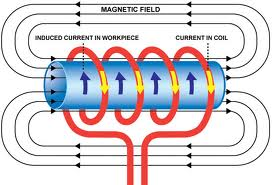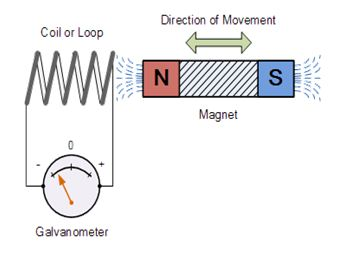# Electromagnetic Induction Study Material for IIT JEE Exam

Electromagnetic induction refers to the production of a voltage or a potential difference across a conductor when it is exposed to a changing magnetic field. The name of Faraday is generally acknowledged with the discovery of induction.

We first discuss the terms which will be used frequently in this topic and later move on to the Faraday Law of electromagnetic Induction.

Flux: Flux is defined as the rate of flow of a property per unit area. For example, the magnitude of a river's current which gives the quantity of water flowing through a cross-section of the river each second is a kind of flux.

Emf: Emf is an abbreviation of electromagnetic force which is the voltage developed by any source of electrical energy such as a battery. It is denoted by E and is measured in volts. The EMF is also given by the rate of change of the magnetic flux:

E = - dφB/ dt,

where E is the electromagnetic force Emf in volts and ΦB is the magnetic flux.Faraday's laws of electromagnetic induction is a basic law of electromagnetism which describes the interaction of a magnetic field with an electric circuit to produce an emf I. It is the prime operating principle of various kinds of motors and generators.

Christian Oersted’s discovery of magnetic field around a current carrying conductor was quite accidental. If a flow of electric current can produce a magnetic field then why can't a Magnetic field produce an electric current? While searching for an answer to this Michel Faraday ended up inventing generators.

For more on electromagnetic induction view the video

We now discuss in detail the Michael Faraday Law:

Relationship between Induced Emf and Flux

In this experiment Faraday took a magnet and a coil and connected a galvanometer across the coil. In the beginning the magnet is at rest so there is no deflection in the galvanometer and hence the needle of galvanometer is at center or zero position. When the magnet is moved toward the coil, the needle of galvanometer deflects in one direction. When the magnet is held stationary at that position, the needle of galvanometer returns back to zero position. Now when the magnet is moved away from the coil , there is some deflection in the needle but in opposite direction and again when the magnet becomes stationary at that point with respect to coil , the needle of galvanometer return back to zero position.

Also if the magnet is held stationary and the coil is moved away and towards the magnet, the galvanometer shows deflection in a similar manner. It is also observed that faster the change in the magnetic field, the greater will be the induced emf or voltage in the coil.

The induced electromotive force in any closed circuit is equal to the negative of the time rate of change of the magnetic flux through the circuit. This version of the Faraday’s law is valid only when the closed circuit is a loop of indefinitely thin wire.## Application of Electromagnetism in Physics (including the Faraday’s Law):

Faraday’s Law is a fundamental law of electromagnetism. This law has widespread applications in various fields including industries, electrical machines etc. some of the major ones are listed below:

• Electrical Transformers

This is a static device which is used for increasing or decreasing thhe voltage or current. It has its applications in generating station, transmission and distribution system. The transformer works on Faraday's law.

• Electrical Generators

The basic working principle of electrical generator is Faraday's law of mutual induction .Electric generator is used to convert mechanical energy into electrical energy.

• Induction Cookers

The Induction cooker also works on principle of mutual induction. When current flows through the coil of copper wire placed below a cooking container, it produces a changing magnetic field. This alternating or changing magnetic field induces an emf and hence the current in the conductive container.

• Electromagnetic Flow Meters

It is used to measure velocity of blood and certain fluids. When a magnetic field is applied to electrically insulating pipe in which conducting fluids are flowing then according to Faraday's law an electromotive force is induced in it. This induced emf is proportional to velocity of fluid flowing.

• Musical Instruments

It is also used in musical instruments like electric guitar, electric violin etc.

Askiitians provides free Study Material - Course Material for IIT JEE Electromagnetic Induction in IIT JEE physics section, with detailed theory and solved problems of electromagnetic induction. The material contains complete explanation of electromagnetic induction for IIT JEE. This important topic takes away at least 2 numerical problems in all engineering entrance exams. Considering this, numerical section is among the most recommended sections. To get an idea about the types of questions asked on electromagnetic induction in IIT JEE, you may refer the Physics Previous Papers.

### Course Features

• Video Lectures
• Revision Notes
• Previous Year Papers
• Mind Map
• Study Planner
• NCERT Solutions
• Discussion Forum
• Test paper with Video Solution

r